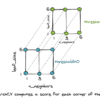# Search results for "normalize"## Scientific Functions in NumPy and SciPy

Python is a general-purpose computation language, but it is very welcomed in scientific computing. It can replace R and Matlab in many cases, thanks to some libraries in the Python ecosystem. In machine learning, we use some mathematical or statistical functions extensively, and often, we will find NumPy and SciPy useful. In the following, we […]## Data Visualization in Python with matplotlib, Seaborn, and Bokeh

Data visualization is an important aspect of all AI and machine learning applications. You can gain key insights into your data through different graphical representations. In this tutorial, we’ll talk about a few options for data visualization in Python. We’ll use the MNIST dataset and the Tensorflow library for number crunching and data manipulation. To […]## Visualizing the vanishing gradient problem

Deep learning was a recent invention. Partially, it is due to improved computation power that allows us to use more layers of perceptrons in a neural network. But at the same time, we can train a deep network only after we know how to work around the vanishing gradient problem. In this tutorial, we visually […]## Using CNN for financial time series prediction

Convolutional neural networks have their roots in image processing. It was first published in LeNet to recognize the MNIST handwritten digits. However, convolutional neural networks are not limited to handling images. In this tutorial, we are going to look at an example of using CNN for time series prediction with an application from financial markets. […]## Face Recognition using Principal Component Analysis

Recent advance in machine learning has made face recognition not a difficult problem. But in the previous, researchers have made various attempts and developed various skills to make computer capable of identifying people. One of the early attempt with moderate success is eigenface, which is based on linear algebra techniques. In this tutorial, we will […]## A Gentle Introduction to Vector Space Models

Vector space models are to consider the relationship between data that are represented by vectors. It is popular in information retrieval systems but also useful for other purposes. Generally, this allows us to compare the similarity of two vectors from a geometric perspective. In this tutorial, we will see what is a vector space model […]## Principal Component Analysis for Visualization

Principal component analysis (PCA) is an unsupervised machine learning technique. Perhaps the most popular use of principal component analysis is dimensionality reduction. Besides using PCA as a data preparation technique, we can also use it to help visualize data. A picture is worth a thousand words. With the data visualized, it is easier for us […]## Modeling Pipeline Optimization With scikit-learn

This tutorial presents two essential concepts in data science and automated learning. One is the machine learning pipeline, and the second is its optimization. These two principles are the key to implementing any successful intelligent system based on machine learning. A machine learning pipeline can be created by putting together a sequence of steps involved […]## A Gentle Introduction to Ensemble Diversity for Machine Learning

Ensemble learning combines the predictions from machine learning models for classification and regression. We pursue using ensemble methods to achieve improved predictive performance, and it is this improvement over any of the contributing models that defines whether an ensemble is good or not. A property that is present in a good ensemble is the diversity […]## How to Combine Predictions for Ensemble Learning

Ensemble methods involve combining the predictions from multiple models. The combination of the predictions is a central part of the ensemble method and depends heavily on the types of models that contribute to the ensemble and the type of prediction problem that is being modeled, such as a classification or regression. Nevertheless, there are common […]